Introduction

ggplot2.dotplot is an easy to use function for making a dot plot with R statistical software using ggplot2 package. The aim of this tutorial, is to show you how to make a dot plot and to personalize the different graphical parameters including main title, axis labels, legend, background and colors. ggplot2.dotplot function is from easyGgplot2 R package. An R script is available in the next section to install the package.

At the end of this tutorial you will be able to draw, with few R code, the following plot :ggplot2.dotplot function is described in detail at the end of this document.

easyGgplot2 R package can be installed as follow :

install.packages("devtools")
library(devtools)
install_github("easyGgplot2", "kassambara")

Load the package using this R code :

library(easyGgplot2)

Data format

The data must be a numeric vector or a data.frame (columns are variables and rows are observations).

ToothGrowth data is used in the following examples.

# create a numeric vector
numVector<-rnorm(100)
##   1.2768 -1.6126 -0.1056  0.4091  0.8999 -0.7300
# data.frame
df <- ToothGrowth
##    len supp dose
## 1  4.2   VC  0.5
## 2 11.5   VC  0.5
## 3  7.3   VC  0.5
## 4  5.8   VC  0.5
## 5  6.4   VC  0.5
## 6 10.0   VC  0.5

ToothGrowth describes the effect of Vitamin C on Tooth growth in Guinea pigs. The response is the length (len) of teeth in each of 10 guinea pigs at each of three dose levels of Vitamin C (0.5, 1, and 2 mg) with each of two delivery methods (orange juice or ascorbic acid).

The data is a data frame with 60 observations on 3 variables. * [,1] len numeric Tooth length. * [,2] supp factor Supplement type (VC or OJ). * [,3] dose numeric Dose in milligrams.

Basic dot plot

# Dot plot from a single numeric vector
ggplot2.dotplot(data=numVector)
# Basic dot plot from the vector "len"
ggplot2.dotplot(data=df, xName='dose',yName='len')
# change dotsize and stack ratio
ggplot2.dotplot(data=df, xName='dose',yName='len',
stackratio=1.5, dotsize=1.2)
# Change the orientation: Horizontal dot plot
ggplot2.dotplot(data=df, xName='dose',yName='len',
orientation="horizontal")
# Dot plot with box plot
ggplot2.dotplot(data=df, xName='dose',yName='len',
# dot plot with notched box plot
ggplot2.dotplot(data=df, xName='dose',yName='len',notch: If TRUE, make a notched box plot. The notch displays a confidence interval around the median which is normally based on the median +/- 1.57 x IQR/sqrt of n. Notches are used to compare groups; if the notches of two boxes do not overlap, this is strong evidence that the medians differ.

Dot plot with mean point

Each dot represents one observation and the mean point corresponds to the mean value of the observations in a given group.

# Dot plot with mean point
ggplot2.dotplot(data=df, xName='dose',yName='len',
meanPointColor="black", meanPointFill="blue")
#Change the dot plot border color
ggplot2.dotplot(data=df, xName='dose',yName='len',
colour="red")Parameters

The arguments that can be used to customize x and y axis are listed below :

Parameters Description
mainTitle the title of the plot
mainTitleFont a vector of length 3 indicating respectively the size, the style (“italic”, “bold”, “bold.italic”) and the color of x and y axis titles. Default value is: mainTitleFont=c(14, “bold”, “black”).
xShowTitle, yShowTitle if TRUE, x and y axis titles will be shown. Set the value to FALSE to hide axis labels. Default values are TRUE.
xtitle, ytitle x and y axis labels. Default values are NULL.
xtitleFont, ytitleFont a vector of length 3 indicating respectively the size, the style and the color of x and y axis titles. Possible values for the style:“plain”, “italic”, “bold”, “bold.italic”. Color can be specified as an hexadecimal code (e.g: “#FFCC00”) or by the name (e.g : “red”, “green”). Default values are xtitleFont=c(14,"bold", "black"), ytitleFont=c(14,"bold", "black").
xlim, ylim limit for the x and y axis. Default values are NULL.
xScale, yScale x and y axis scales. Possible values : c(“none”, “log2”, “log10”). e.g: yScale=“log2”. Default values are NULL.
xShowTickLabel, yShowTickLabel if TRUE, x and y axis tick mark labels will be shown. Default values are TRUE.
xTickLabelFont, yTickLabelFont a vector of length 3 indicating respectively the size, the style and the color of x and y axis tick label fonts. Default value are xTickLabelFont=c(12, "bold", "black"), yTickLabelFont=c(12, "bold", "black").
xtickLabelRotation, ytickLabelRotation Rotation angle of x and y axis tick labels. Default value are 0.
hideAxisTicks if TRUE, x and y axis ticks are hidden. Default value is FALSE.
axisLine a vector of length 3 indicating respectively the size, the line type and the color of axis lines. Default value is c(0.5, "solid", "#E5E5E5").

Main title and axis labels

# Change main title and axis titles
ggplot2.dotplot(data=df, xName='dose',yName='len',
mainTitle="Plot of length \n by dose",
xtitle="Dose (mg)", ytitle="Length")
# Customize title styles. Possible values for the font style :
# 'plain', 'italic', 'bold', 'bold.italic'.
ggplot2.dotplot(data=df, xName='dose',yName='len',
xtitle="Dose (mg)", ytitle="Length",
mainTitle="Plot of length \n by dose",
mainTitleFont=c(14,"bold.italic", "red"),
xtitleFont=c(14,"bold", "#993333"),
ytitleFont=c(14,"bold", "#993333"))
# Hide x an y axis titles
ggplot2.dotplot(data=df, xName='dose',yName='len',
xShowTitle=FALSE, yShowTitle=FALSE)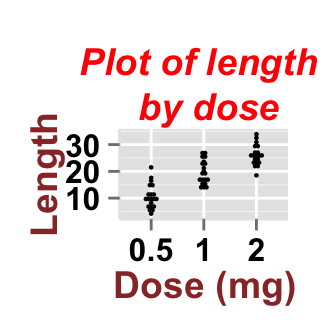Axis ticks

# Axis ticks labels and orientaion
ggplot2.dotplot(data=df, xName='dose',yName='len',
xShowTitle=FALSE, yShowTitle=FALSE,
xTickLabelFont=c(14,"bold", "#993333"),
yTickLabelFont=c(14,"bold", "#993333"),
xtickLabelRotation=45, ytickLabelRotation=45)
# Hide axis tick labels
ggplot2.dotplot(data=df, xName='dose',yName='len',
xShowTitle=FALSE, yShowTitle=FALSE,
xShowTickLabel=FALSE, yShowTickLabel=FALSE)
# Hide axis ticks
ggplot2.dotplot(data=df, xName='dose',yName='len',
xShowTitle=FALSE, yShowTitle=FALSE,
xShowTickLabel=FALSE,yShowTickLabel=FALSE,
hideAxisTicks=TRUE)
# AxisLine : a vector of length 3 indicating the size,
#the line type and the color of axis lines
ggplot2.dotplot(data=df, xName='dose',yName='len',
axisLine=c(1, "solid", "darkblue"))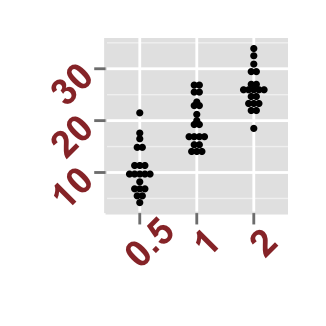Background and colors

Change dot plot background and fill colors

# change background color to "white". Default is "gray"
ggplot2.dotplot(data=df, xName='dose',yName='len',
backgroundColor="white")
# change background color to "lightblue" and grid color to "white"
ggplot2.dotplot(data=df, xName='dose',yName='len',
backgroundColor="lightblue", gridColor="white")
# Change plot fill color
ggplot2.dotplot(data=df, xName='dose',yName='len',
# remove grid; remove top and right borders around the plot;
# change  axis lines
ggplot2.dotplot(data=df, xName='dose',yName='len',
removePanelGrid=TRUE,removePanelBorder=TRUE,
axisLine=c(0.5, "solid", "black"))Change dot plot color according to the group

Colors can be specified as a hexadecimal RGB triplet, such as "#FFCC00" or by names (e.g : "red" ). You can also use other color scales, such as ones taken from the RColorBrewer package. The different color systems available in R have been described in detail here.

To change dot plot color according to the group, you have to specify the name of the data column containing the groups using the argument groupName. Use the argument groupColors, to specify colors by hexadecimal code or by name. In this case, the length of groupColors should be the same as the number of the groups. Use the argument brewerPalette, to specify colors using RColorBrewerpalette.

# Color the dot plot accoording to the groupName "dose"
ggplot2.dotplot(data=df, xName='dose',yName='len', groupName='dose')
#Change group colors using hexadecimal colors
ggplot2.dotplot(data=df, xName='dose',yName='len', groupName='dose',
groupColors=c('#999999','#E69F00','#56B4E9'))
#Change group colors using brewer palette: "Paired"
ggplot2.dotplot(data=df, xName='dose',yName='len', groupName='dose',
brewerPalette="Paired")Color can also be changed by using names as follow :

#Change group colors using color names
ggplot2.dotplot(data=df, xName='dose',yName='len',
groupName='dose',
groupColors=c('aquamarine3','chartreuse1','goldenrod1'))

Legend

Legend position

# Change the legend position to "top"
# (possible values: "left","top", "right", "bottom")
ggplot2.dotplot(data=df, xName='dose',yName='len', groupName='dose',
legendPosition="top")
# legendPosition can be also a numeric vector c(x, y)
ggplot2.dotplot(data=df, xName='dose',yName='len', groupName='dose',
legendPosition=c(0.8,0.2))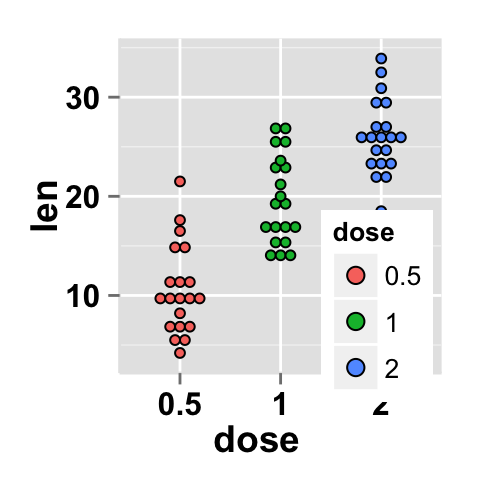It is also possible to position the legend inside the plotting area. You have to indicate the x, y coordinates of legend box. x and y values must be between 0 and 1. c(0,0) corresponds to "bottom left" and c(1,1) corresponds to "top right" position.

Legend background color, title and text font styles

# Change legend background color, title and text font styles
ggplot2.dotplot(data=df, xName='dose',yName='len', groupName='dose',
# legendTitleFont=c(size, style, color)
legendTitle="Dose (mg)",
legendTitleFont=c(10, "bold", "blue"),
#legendTextFont=c(size, style, color)
legendTextFont=c(10, "bold.italic", "red"),
# legendBackground: c(fill, lineSize, lineType, lineColor)
legendBackground=c("lightblue", 0.5, "solid", "darkblue" )
)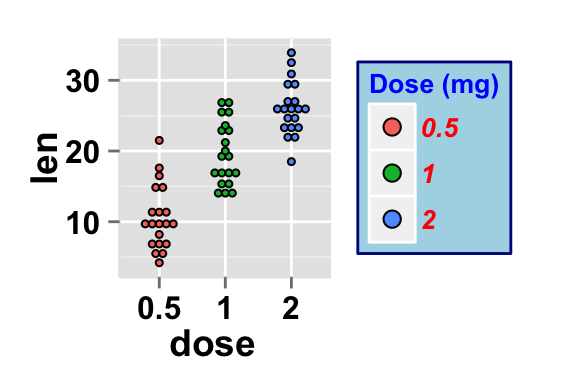Change the order of items in the legend, remove plot legend

# Change the order of items in the legend
# legendItemOrder : character vector indicating
# the order of items in the legends.
ggplot2.dotplot(data=df, xName='dose',yName='len', groupName='dose',
legendItemOrder=c("2", "1", "0.5"))

# Remove plot legend
ggplot2.dotplot(data=df, xName='dose',yName='len', groupName='dose',
showLegend=FALSE)Axis scales

Possible values for y axis scale are “none”, “log2” and log10. Default value is “none”.

# Change y axis limit
ggplot2.dotplot(data=df, xName='dose',yName='len', groupName='dose',
ylim=c(0,50))
# y Log scale. Possible values="none", "log2" and "log10"
ggplot2.dotplot(data=df, xName='dose',yName='len', groupName='dose',
yScale="log2")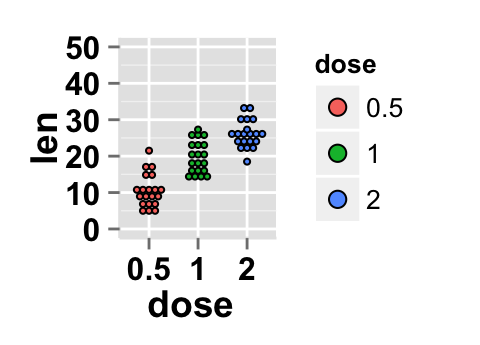Create a customized plots with few R code

#Customized dot plot
ggplot2.dotplot(data=df, xName='dose',yName='len', groupName='dose',
groupColors=c('#999999','#E69F00','#56B4E9'), showLegend=FALSE,
backgroundColor="white", xtitle="Dose (mg)", ytitle="length",
mainTitle="Plot of length \n by dose")
# Customized dot plot with notched box plot
# Remove grid; Remove Top and right border around the plot
ggplot2.dotplot(data=df, xName='dose',yName='len', groupName='dose',
groupColors=c('#999999','#E69F00','#56B4E9'), showLegend=FALSE,
backgroundColor="white", xtitle="Dose (mg)", ytitle="length",
mainTitle="Plot of length \n by dose",
removePanelGrid=TRUE,removePanelBorder=TRUE,
axisLine=c(0.5, "solid", "black"))# Customized dot plot, add box plot, pink fill color.
ggplot2.dotplot(data=df, xName='dose',yName='len', groupName='dose',
groupColors=c('#999999','#E69F00','#56B4E9'), showLegend=FALSE,
backgroundColor="white", xtitle="Dose (mg)", ytitle="length",
mainTitle="Plot of length \n by dose",
# Customized dot plot, add box plot,
#fill box plot accoording to the groups.
ggplot2.dotplot(data=df, xName='dose',yName='len', groupName='dose',
groupColors=c('#999999','#E69F00','#56B4E9'), showLegend=FALSE,
backgroundColor="white", xtitle="Dose (mg)", ytitle="length",
mainTitle="Plot of length \n by dose",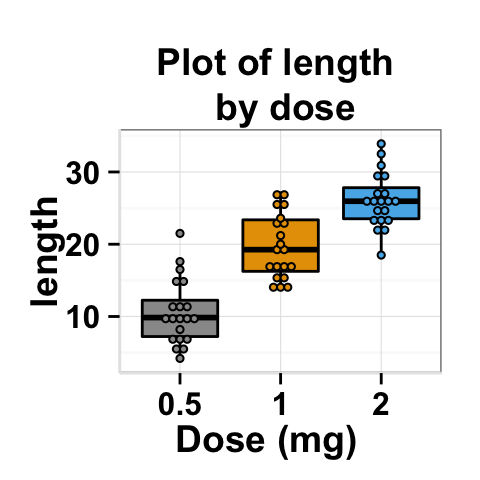By default, box plot fill color is “white”. To change the fill color with the argument groupColors, set boxplotFill value to NULL.

Dot plot with multiple groups

The data format is:

##     len supp dose
## 1   4.2   VC  0.5
## 2  11.5   VC  0.5
## 3   7.3   VC  0.5
## 11 16.5   VC  1.0
## 12 16.5   VC  1.0
## 13 15.2   VC  1.0
## 14 17.3   VC  1.0
## 15 22.5   VC  1.0
## 21 23.6   VC  2.0
## 22 18.5   VC  2.0
## 23 33.9   VC  2.0
## 24 25.5   VC  2.0
## 31 15.2   OJ  0.5
## 32 21.5   OJ  0.5
## 33 17.6   OJ  0.5
## 34  9.7   OJ  0.5
## 41 19.7   OJ  1.0
## 42 23.3   OJ  1.0
## 43 23.6   OJ  1.0
## 44 26.4   OJ  1.0
## 51 25.5   OJ  2.0
## 52 26.4   OJ  2.0
## 53 22.4   OJ  2.0
## 54 24.5   OJ  2.0
# plot of variable 'len' by xName 'dose'. The plot is colored by the groupName 'supp'
# position = interval between dot plot of the same group
ggplot2.dotplot(data=df, xName='dose',yName='len', groupName='supp',
position=position_dodge(0.8),
backgroundColor="white",groupColors=c('#999999','#E69F00')
)

# Change the interval between dotplot of the same group :
# position=position_dodge(0); overlap
ggplot2.dotplot(data=df, xName='dose',yName='len', groupName='supp',
position=position_dodge(0.0),
backgroundColor="white", groupColors=c('#999999','#E69F00'),Facet : split a plot into a matrix of panels

The facet approach splits a plot into a matrix of panels. Each panel shows a different subset of the data.

Facet with one variable

#Facet according to the supp variable
ggplot2.dotplot(data=df, xName='dose',yName='len', groupName='supp',
legendPosition="top",
faceting=TRUE, facetingVarNames="supp")
# Change the direction. possible values are "vertical", horizontal".
# default is vertical.
ggplot2.dotplot(data=df, xName='dose',yName='len', groupName='supp',
legendPosition="top",
faceting=TRUE, facetingVarNames="supp",
facetingDirection="horizontal")Facet with two variables

# Facet by two variables: dose and supp.
# Rows are dose and columns are supp
ggplot2.dotplot(data=df, xName='dose',yName='len', groupName='supp',
legendPosition="top", faceting=TRUE,
facetingVarNames=c("dose","supp"))
# Facet by two variables: reverse the order of the 2 variables
# Rows are supp and columns are dose
ggplot2.dotplot(data=df, xName='dose',yName='len', groupName='supp',
legendPosition="top", faceting=TRUE,
facetingVarNames=c("supp", "dose"))Facet scales

By default, all the panels have the same scale (facetingScales="fixed"). They can be made independent, by setting scales to free, free_x, or free_y.

# Facet with free scales
ggplot2.dotplot(data=df, xName='dose',yName='len',
groupName='supp', legendPosition="top",
faceting=TRUE, facetingVarNames=c("dose", "supp"),
facetingScales="free")As you can see in the above plot, y axis have different scales in the different panels.

Facet label apperance

# Change facet text font. Possible values for the font style:
#'plain', 'italic', 'bold', 'bold.italic'.
ggplot2.dotplot(data=df, xName='dose',yName='len', groupName='supp',
legendPosition="top",
faceting=TRUE, facetingVarNames=c("dose","supp"),
facetingFont=c(12, 'bold.italic', "red"))
# Change the apperance of the rectangle around facet label
ggplot2.dotplot(data=df, xName='dose',yName='len', groupName='supp',
legendPosition="top",
faceting=TRUE, facetingVarNames=c("dose","supp"),
facetingRect=list(background="white", lineType="solid",
lineColor="black", lineSize=1.5)
)ggplot2.dotplot function

Description

Plot easily a dot plot plot with R package easyGgplot2.

usage

ggplot2.dotplot(data, xName=NULL, yName=NULL, groupName=NULL,
position=position_dodge(0.8),
meanPointColor="black", meanPointFill="blue",
boxplotColor="black", boxplotLineWeight=0.5,
groupColors=NULL, brewerPalette=NULL,...)

Arguments

Arguments Descriptions
data data.frame or a numeric vector. Columns are variables and rows are observations.
xName The name of column containing x variable (i.e groups). Default value is NULL.
yName The name of column containing y variable. If yName=NULL, data should be a numeric vector.
groupName The name of column containing group variable. This variable is used to color plot according to the group.
position The position adjustment to use for overlapping points. Default value is position_dodge(0.8).
addMean if TRUE, the mean point is added on the plot for each group. Default value is FALSE.
meanPointShape, meanPointSize The shape and size of mean point.
meanPointColor border color of the mean point. Default value is “black”.
meanPointFill Fill color of mean point. This parameter is used only when meanPointShape=21 to 25. Default value is “blue”
addBoxplot If TRUE, boxplot is added on the dotplot. Default value is FALSE.
boxplotFill Fill color of the boxplot. Default value is white.
boxplotColor Boxplot line color. Default value is black.
boxplotLineWeight Boxplot line weight. Default value is 0.5.
groupColors Color of groups. groupColors should have the same length as groups.
brewerPalette This can be also used to indicate group colors. In this case the parameter groupColors should be NULL. e.g: brewerPalette=“Paired”.
…. Other arguments passed on to ggplot2.customize custom function or to geom_dotplot functions from ggplot2 package.

The other arguments which can be used are described at this link : ggplot2 customize. They are used to customize the plot (axis, title, background, color, legend, ….) generated using ggplot2 or easyGgplot2 R package.

Examples

df <- ToothGrowth
ggplot2.dotplot(data=df, xName='dose',yName='len',
mainTitle="Plot of length according\n to the dose",
xtitle="Dose (mg)", ytitle="Length")
#Or use this
plot<-ggplot2.dotplot(data=df, xName='dose',yName='len')
plot<-ggplot2.customize(plot, mainTitle="Plot of length according\n to the dose",
xtitle="Dose (mg)", ytitle="Length")
print(plot)

Easy ggplot2 ebook

Note that an eBook is available on easyGgplot2 package here.

September 2014 : First edition.

Licence : This document is under creative commons licence (http://creativecommons.org/licenses/by-nc-sa/3.0/).

Infos

This analysis was performed using R (ver. 3.1.0), easyGgplot2 (ver 1.0.0) and ggplot2 (ver 1.0.0).